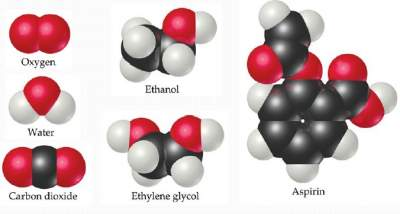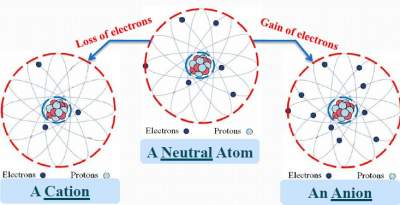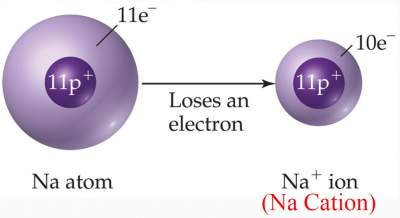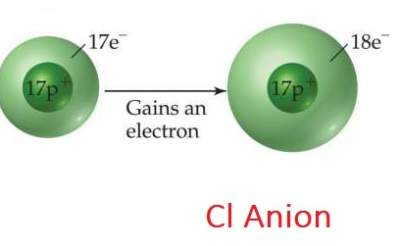# Atoms Combine to Form Molecules & Ion

## Combine Atoms into Molecules & Ions

### Molecule

It is an assembly of 2 or more atoms (of the sameor differentelement(s))tightly bound together,
and behave as a SINGLE unit with its own properties. Examples are shown below:Note that most elements in the periodic table exist as SINGLE atoms but
there are a few that exist only in "diatomic" molecules,
such as: O2,H2, F2, Cl2, N2, Br2, and I2

#### Types of Molecules

1. Molecular: Where two or more non-metallic atomes are bonded to each other
2. Ionic: Where two or more metallic & non-metallic atoms are bonded to each other
Examples of Molecular: H2O, H2O2, HCl
Examples of Ionic: NaCl, FeO3, ZnS

### Ions and Ionic Compounds

Protons are entrapped inside an atom; their number CANNOT Change in a chemical reaction.
Only number of electrons can change during a chemical reaction. The loss and gain of electrons is illustrated below:#### Examples### Ions and Ionic Compounds: Rules

1. Metals tend to lose electrons forming Cations
2. Non-metals tend to gain electrons forming Anions
3. Ions such as Na+ and Cl are called "Mono atomic ions"
4. "Poly atomic ions" are atoms joined in a form of molecule, but with a positive or negative net charge.
Example: NO3, SO42−
5. Chemical properties of ions are totally different from their corresponding atoms because of the difference in charge.
Example: Na+ & Cl ions are different from Na, Cl atoms.

#### Example

Assume you need to find out the number of protons and electrons that Se2− ion possess.
According to the periodic table, Se is an element with an atomic number of 34, which means # protons = 34.
Since charge = (# protons) − (# electrons), hence , -2 = 34 –(# electrons), which means (# electrons) = 36.

### Exercise: Chemical Symbol and Atomic Mass

Exercise on Chemical Symbol and Atomic Mass

Check your answers here: Solutions to the Exercise on Chemical Symbol and Atomic Mass

### Predicting ionic charge

Atoms lose or gain electrons in order to reach the same number of electrons of the closest "Noble Gas".

#### Example 1

Na has an atomic number of 11. It tends to lose an electron (forming Na+) with 10 electrons = atomic number of Ne (The closest inert gas).

#### Example 2

Cl has an atomic number of 17. It tends to gain an electron (forming Cl) with 18 electrons = atomic number of Ar (The closest inert gas).

#### Example 3

Ba has an atomic number of 56. It tends to lose two electrons (forming Ba 2+) with 54 electrons = atomic number of Xe (The closest inert gas).

### Formation of an Ionic Compound

Electrons lost by atoms to form cations are gained by other atoms to form anions in the same ionic compound.
Ionic Compounds Are NEUTRAL.

#### Example 1

Na+ & Cl → NaCl
Mg2+ & 2Cl → MgCl2

#### Example 2

If the positive charge on ion A ≠ negative charge on ion B. For example: Mg2+ and N3−
In this case the following rule applies:
Number of Mg ions = Charge on N ions AND Number of N ions = Charge on Mg ions
3 × Mg2+ = 6+ charges and 2 × N3− = 6 − charges ⇔ Mg3N2 has ZERO net charge.

### Exercise: Formation of an Ionic Compound

Exercise on Formation of an Ionic Compounds & Empirical Formula

Check your answers here: Solutions to the Exercise on Formation of an Ionic Compounds & Empirical Formula

### Exercise: Formation of Ionic Compounds vs. Molecular Compounds

Exercise on Formation of Ionic Compounds vs. Molecular Compounds

Check your answers here: Solutions to the Exercise on Formation of Ionic Compounds vs. Molecular Compounds